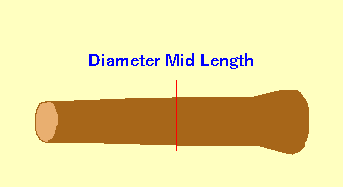Spike's Calculators

# Swedish Log Volume Calculation Method Two

Swedish Log Volume Calculators: Method One - Method Two - Method Three"Measuring of Roundwood" as recommended by the Timber Measurement Council

Measuring log volume using mid-point diameter measurement
Mid-point measurement may be used on all tree species, regardless of diameter and length.
Diameter measurements are measured under the bark.

##### Formula used:
`V = π ÷ 4 * L * Dm²`
where:
V = the log volume in cubic metres
Dm = the mid-point diameter

### Using Mid-Point Diameter

Length of Log m
Mid-length Diameter cm

#### Results:

 Log Volume m³

#### Calculation

1. the log length in metres
2. the diameter measured at the mid-length of the log